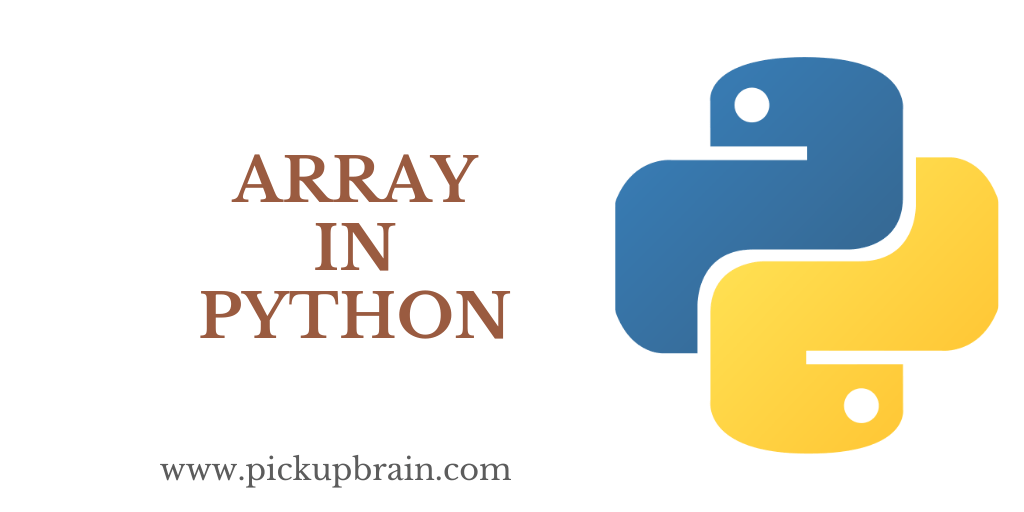# Array in PythonAn array in python is a data structure that contain a group of elements. These are use in computer program to organize data so that related set of value can easily stored or searched.

It is a collection of elements having the same data type and always start from index value and index start from 0 to 1.

It doesn’t have a fixed size which means it can be expand it or shrink it.

We can declare it as below:

Here type of array is an integer with size 5 which means it can store 5 elements. We can access each element by using an index.

## Implementation of Array

In Python, array can created by importing array module.

While working with array we need to specify two things:

• Type of the array because in array size should be same.
• Values

For type of array we can refer this chart:

So let’s create an array of integer

```#Creating an integer array

from array import *

arr = array('i',[10,50,60,80,40])

print(arr)

>>>Output
array('i', [10, 50, 60, 80, 40])
```

### Accessing an element from Python Array

In python, an index operator for accessing an element from the array.

Let’s say we need to access 3rd element from the list for that we will simply write “a = ” as the index in python starts from ‘0’.

```#Accessing an element from an array

from array import *

arr = array('i',[10,50,60,80,40])

print(arr)

>>>Output
60
```

### Updating an Array in Python

To update elements in an array, reassign a new value to the specified index in which we want to update.

Suppose we want to update 25 instead of 50 in the above array.

For that simply write.

```#Updating an array

from array import *

arr = array('i',[10,50,60,80,40])

arr = 25

print(arr)

>>>Output
array('i', [10, 25, 60, 80, 40])
```

### Length of an Array

In python, the len() method is used to find the length of an array.

```#Length of an Array
from array import *

arr = array('i',[10,50,60,80,40])

a= len(arr)

print(a)

>>>Output
5
```

### Deleting an Element from Array

To delete array elements from the array we can use pop() method to delete.

```#deleting an element from an array
from array import *

arr = array('i',[10,50,60,80,40])

arr.pop(4)

print(arr)

>>>Output
array('i', [10, 50, 60, 80])
```

### Append element in an Array

To add element in an existing array we can use append() method for adding the elements in python.

```#append an element from an array
from array import *

arr = array('i',[10,50,60,80,40])

arr.append(35)

print(arr)

>>> Output
array('i', [10, 50, 60, 80, 40, 35])
```

### Inserting an element in Array

To insert value at any index of the array we will use the insert() method.

Here, the first argument is for the index, and the second argument is for value.

```#inserting an element in array
from array import *

arr = array('i',[10,50,60,80,40])

arr.insert(2,90)

print(arr)

>>>Output
array('i', [10, 50, 90, 60, 80, 40])
```

### Extend Array in Python

An array value can be extended with more than one value by using extend() method.

```#Extend array in python
from array import *

arr1 = array('i',[10,50,60,80,40])

arr2 = array('i',[100,900,800,500,200])

arr1.extend(arr2)
print(arr1)

>>>Output
array('i', [10, 50, 60, 80, 40, 100, 900, 800, 500, 200])
```

## Important Points of Array

• Array can store only one type of data.
• Size of array is not fix. It can increase or decrease their size dynamically.
• Array and List are not the same as array can store elements of same data type but the list can store elements of multiple data type.
• It uses less memory than List.